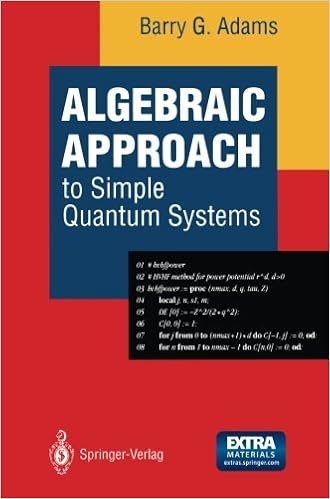This e-book offers an creation to using algebraic equipment and sym­ bolic computation for easy quantum platforms with purposes to massive order perturbation concept. it's the first booklet to combine Lie algebras, algebraic perturbation thought and symbolic computation in a kind appropriate for college kids and researchers in theoretical and computational chemistry and is with ease divided into elements. the 1st half, Chapters 1 to six, offers a pedagogical creation to the \$64000 Lie algebras so(3), so(2,1), so(4) and so(4,2) wanted for the learn of easy quantum structures comparable to the D-dimensional hydrogen atom and harmonic oscillator. This fabric is appropriate for complex undergraduate and starting graduate scholars. Of specific value is using so(2,1) in bankruptcy four as a spectrum producing algebra for numerous vital structures akin to the non-relativistic hydrogen atom and the relativistic Klein-Gordon and Dirac equations. This process offers an engaging and demanding replacement to the standard textbook method utilizing sequence strategies of differential equations.

Similar quantum theory books

A Mathematical Introduction to Conformal Field Theory

The 1st a part of this publication offers an in depth, self-contained and mathematically rigorous exposition of classical conformal symmetry in n dimensions and its quantization in dimensions. particularly, the conformal teams are made up our minds and the looks of the Virasoro algebra within the context of the quantization of two-dimensional conformal symmetry is defined through the class of imperative extensions of Lie algebras and teams.

The Physics of Atoms and Molecules

This ebook is superb for a 1st 12 months graduate direction on Atomic and Molecular physics. The preliminary sections conceal QM in pretty much as good and concise a way as i have ever noticeable. The assurance of perturbation concept can also be very transparent. After that the ebook concentrates on Atomic and Molecular themes like nice constitution, Hyperfine strucutre, Hartree-Fock, and a really great part on Atomic collision physics.

Quantum Invariants of Knots and 3-Manifolds

This monograph, now in its moment revised version, presents a scientific therapy of topological quantum box theories in 3 dimensions, encouraged by means of the invention of the Jones polynomial of knots, the Witten-Chern-Simons box idea, and the speculation of quantum teams. the writer, one of many prime specialists within the topic, offers a rigorous and self-contained exposition of basic algebraic and topological techniques that emerged during this conception

Additional resources for Algebraic Approach to Simple Quantum Systems: With Applications to Perturbation Theory

Example text

Selection Rules for a Vector Operator 31 operator. 24) also show that rand p are vector operators. We now obtain several identities relating J and V which will be used to determine matrix elements of components of V. 25) J XV Since J1 V1 +V X J = 2iV. 26) = V1 J1 and similarly for the other components, JoV = VoJ. 28) which can be expressed in vector form as [J V, J] 0 = O. 30) The following three identities are also important: [J2, V] = i(V X J - J X V), [J2, J X V] = 2i(J 2V - (J V)J), [J2, [J2, V]] = 2(J 2V - 2(J V)J + V J2).

They have the same structure and importance as the product rule for differentiation does in the calculus: in fact, defining D A(B) = [A, B] , the first rule becomes D A(BC) = BDA(C) + DA(B)C. The following two rules are often useful for moving functions of an operator inside or outside a commutator: f(A)[g(A), BJ = [g(A), f(A)B], [A, g(B)]J(B) = [Af(B),g(B)]. 4) Chapter 2. Commutator Gymnastics 18 Here we assume that a function of an operator is a polynomial or a formal power series in the operator without regard to the convergence of the power series.

4. 14) Since the eigenvalues of an hermitian operator are real it follows that A and m are real. /JAmI12 > 0, O. /JAm, (J 2 IILtPAml12 = (tPAm, (J 2 - = IBAml2 ~ J2 - J; + J3 and LJ+ = o. J2 - J; - J3 J; - J3 )tPAm) = A - m(m + 1), Ji + J3 )tPAm) = A - m(m - 1). Therefore IAAmI 2 =A-m(m+l) ~ 0, IBAm I2 =A-m(m-l) ~ O. 16) Adding these results together gives m 2 ~ A which means that the eigenvalue spectrum of J3 is bounded above and below (case (4)) and the only unirreps are the finite dimensional ones.